Home / Expert Answers / Chemistry / at-1-bar-how-much-energy-is-required-to-heat-63-0-mathrm-g-mathrm-h-2-mathrm-o-mathrm-pa885

# (Solved): At 1 bar, how much energy is required to heat $$63.0 \mathrm{~g} \mathrm{H}_{2} \mathrm{O}(\mathrm ...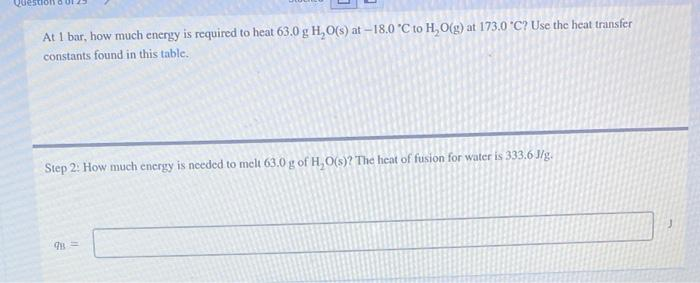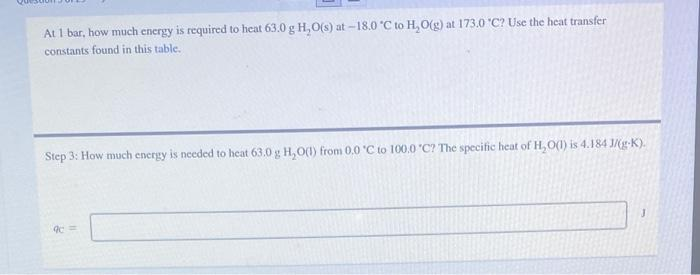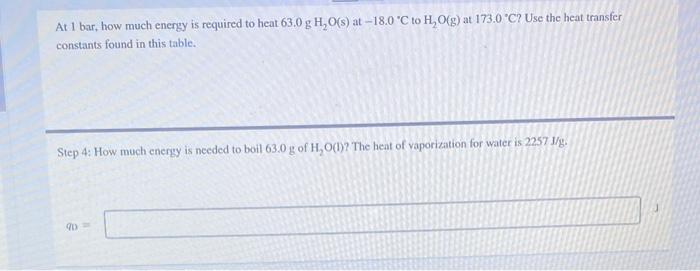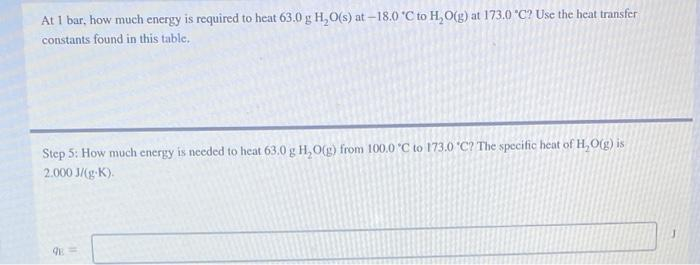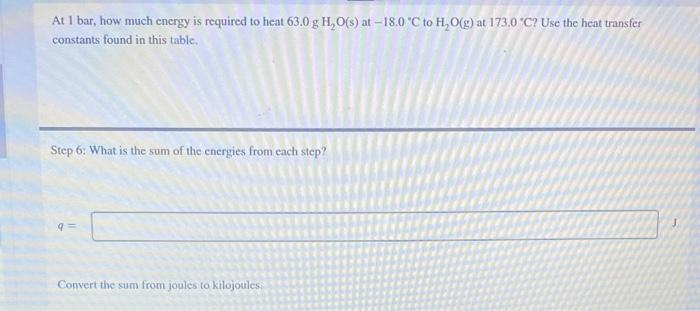At 1 bar, how much energy is required to heat \( 63.0 \mathrm{~g} \mathrm{H}_{2} \mathrm{O}(\mathrm{s})$$ at $$-18.0^{\circ} \mathrm{C}$$ to $$\mathrm{H}_{2} \mathrm{O}(\mathrm{g})$$ at $$173.0^{\circ} \mathrm{C}$$ ? Use the heat transfer constants found in this table. Step 2: How much energy is needed to melt $$63.0 \mathrm{~g} \mathrm{of} \mathrm{H}_{2} \mathrm{O}(\mathrm{s})$$ ? The heat of fusion for water is $$333.6 \mathrm{~J} / \mathrm{g}$$. At I bar, how much energy is required to heat $$63.0 \mathrm{~g} \mathrm{H} \mathrm{O}(\mathrm{s})$$ at $$-18.0^{\circ} \mathrm{C}$$ to $$\mathrm{H}_{2} \mathrm{O}(\mathrm{g})$$ at $$173.0^{\circ} \mathrm{C}$$ ? Use the heat transfer constants found in this table. Step 3: How much energy is needed to heat $$63.0 \mathrm{~g} \mathrm{H}_{2} \mathrm{O}(\mathrm{I})$$ from $$0.0^{\circ} \mathrm{C}$$ to $$100.0$$ " $$\mathrm{C}$$ ? The specific heat of $$\mathrm{H}_{2} \mathrm{O}(1)$$ is $$4.184 \mathrm{~J} /(\mathrm{g} \cdot \mathrm{K})$$. At 1 bar, how much energy is required to heat $$63.0 \mathrm{~g} \mathrm{H} \mathrm{H}_{2} \mathrm{O}(\mathrm{s})$$ at $$-18.0^{\circ} \mathrm{C}$$ to $$\mathrm{H}_{2} \mathrm{O}(\mathrm{g})$$ at $$173.0^{\circ} \mathrm{C}$$ ? Use the heat transfer constants found in this table. Step 4: How much energy is needed to boil $$63.0 \mathrm{~g} \mathrm{of} \mathrm{H}_{2} \mathrm{O}(\mathrm{l})$$ ? The heat of vaporization for water is $$2257 \mathrm{~J} / \mathrm{g}$$. At 1 bar, how much energy is required to heat $$63.0 \mathrm{~g} \mathrm{H} \mathrm{O}(\mathrm{s})$$ at $$-18.0^{\circ} \mathrm{C}$$ to $$\mathrm{H}_{2} \mathrm{O}(\mathrm{g})$$ at $$173.0{ }^{\circ} \mathrm{C}$$ ? Use the heat transfer constants found in this tablc. Step 5: How much energy is needed to heat $$63.0 \mathrm{~g} \mathrm{H}_{2} \mathrm{O}(\mathrm{g})$$ from $$100.0^{\circ} \mathrm{C}$$ to $$173.0^{\circ} \mathrm{C}$$ ? The specific heat of $$\mathrm{H}_{2} \mathrm{O}(\mathrm{g})$$ is $$2.000 \mathrm{~J} /(\mathrm{g} \cdot \mathrm{K})$$ At 1 bar, how much energy is required to heat $$63.0 \mathrm{~g} \mathrm{H}_{2} \mathrm{O}(\mathrm{s})$$ at $$-18.0^{\circ} \mathrm{C}$$ to $$\mathrm{H}_{2} \mathrm{O}(\mathrm{g})$$ at $$173.0{ }^{\circ} \mathrm{C}$$ ? Use the heat transfer constants found in this table. Step 6: What is the sum of the energies from each step? Convert the sum from joules to kilojoules:

We have an Answer from Expert